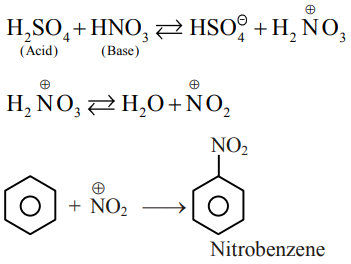# Benzene on nitration gives nitrobenzene in presence`
Question:

Benzene on nitration gives nitrobenzene in presence of $\mathrm{HNO}_{3}$ and $\mathrm{H}_{2} \mathrm{SO}_{4}$ mixture, where :

1. both $\mathrm{H}_{2} \mathrm{SO}_{4}$ and $\mathrm{HNO}_{3}$ act as a bases

2. $\mathrm{HNO}_{3}$ acts as an acid and $\mathrm{H}_{2} \mathrm{SO}_{4}$ acts as a base

3. both $\mathrm{H}_{2} \mathrm{SO}_{4}$ and $\mathrm{HNO}_{3}$ act as an acids

4. $\mathrm{HNO}_{3}$ acts as a base and $\mathrm{H}_{2} \mathrm{SO}_{4}$ acts as an acid

Correct Option: , 4

Solution:

Reagent for nitration of Benzene